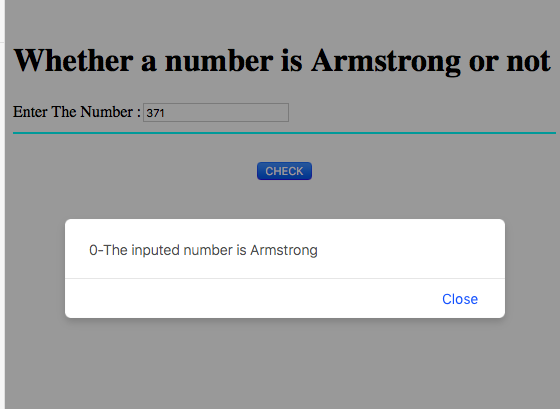# JavaScript code to check number is Armstrong or not

In this JavaScript code, we are going to check whether a given number is Armstrong number or not.
Submitted by Aleesha Ali, on March 28, 2018

## Armstrong number

A number is said to be Armstrong when its value is equal to its sum of cube of digits.

Example:

```    Input:
Number: 153

Output:
It is a prime number

Explanation:
153 = (1*1*1) + (5*5*5) + (3*3*3) = 1+125+27 = 153
Here, number (153) is equal to its sum of the digits cube (153).
```

### JavaScript code to check whether a Number is Armstrong or Not

```<script>
function Armstrong()
{
var flag,number,remainder,addition = 0;
number = Number(document.getElementById("N").value);

flag = number;
while(number > 0)
{
remainder = number%10;
number = parseInt(number/10);
}

{
window.alert("-The inputed number is Armstrong");
}
else
{
window.alert("-The inputed number is not Armstrong");
}
}
</script>
```

Calling JavaScript function in HTML (HTML, JS Code)

```<html>
<script>
function Armstrong()
{
var flag,number,remainder,addition = 0;
number = Number(document.getElementById("N").value);

flag = number;
while(number > 0)
{
remainder = number%10;
number = parseInt(number/10);
}

{
window.alert("-The inputed number is Armstrong");
}
else
{
window.alert("-The inputed number is not Armstrong");
}
}
</script>
<body>
<br>
<h1>Whether a number is Armstrong or not</h1>
Enter The Number :<input type="text" name="n" id = "N"/>
<hr color="cyan">
<br>
<center><button onClick="Armstrong()">CHECK</button>
</body>
</html>
```

OutputWhat's New (MCQs)

Top Interview Coding Problems/Challenges!

Languages: » C » C++ » C++ STL » Java » Data Structure » C#.Net » Android » Kotlin » SQL
Web Technologies: » PHP » Python » JavaScript » CSS » Ajax » Node.js » Web programming/HTML
Solved programs: » C » C++ » DS » Java » C#
Aptitude que. & ans.: » C » C++ » Java » DBMS
Interview que. & ans.: » C » Embedded C » Java » SEO » HR
CS Subjects: » CS Basics » O.S. » Networks » DBMS » Embedded Systems » Cloud Computing
» Machine learning » CS Organizations » Linux » DOS
More: » Articles » Puzzles » News/Updates

© https://www.includehelp.com some rights reserved.